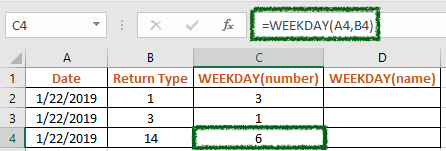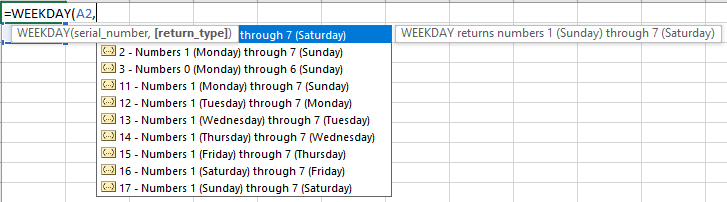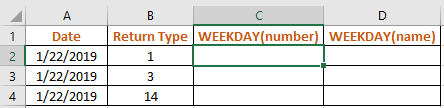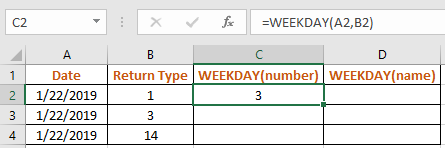# How to use the WEEKDAY function in ExcelIn this article, we will learn How to use the WEEKDAY function in Excel.

What is a weekday ?

Usually we recall days with either date value or the weekday value. For example 4th Sunday of October, Sunday is the weekday associated with the date. There are 7 weekdays in a week. With each date having its own weekday. Weekdays : Sunday, Monday, Tuesday, Wednesday, Thursday, Friday and Saturday.

In Excel, dates are considered as numbers in Excel whereas weekdays are text values. So WEEKDAY function returns the weekday of the input date value. Let's learn WEEKDAY function syntax and an example to illustrate the function usage.

WEEKDAY Function in Excel

The WEEKDAY function takes the date value ( as number ) in the argument and return number corresponding to the weekday.

Syntax:

 =WEEKDAY( date_value, [Return Ttype])

date_value : date value as number or using cell reference

The function has a different return_type (optional) argument. Learn return_type argument from the table.#### Return Type

 Return Type Result Weekday Start Weekday End - 1-7 Sunday Saturday 1 1-7 Sunday Saturday 2 1-7 Monday Sunday 3 0-6 Monday Sunday 11 1-7 Monday Sunday 12 1-7 Tuesday Monday 13 1-7 Wednesday Tuesday 14 1-7 Thursday Wednesday 15 1-7 Friday Thursday 16 1-7 Saturday Friday 17 1-7 Sunday Saturday

Note: the WEEKDAY function will return a value even when the date is empty. Take care to trap this result if blank cells are possible in the list.

Example :

All of these might be confusing to understand. Let's understand how to use the function using an example. Here we have Dates and Return Types as arguments for the Function.Use the formula:

 =WEEKDAY(A1,B2)The formula returned 3. Tuesday is for 3 when return type is 1 i.e. Sunday - Saturday

Copy the formula in other cells using Shortcutkey Ctrl + D.Weekday number 1 when the return type is 3. The number represents Tuesday.

Weekday number 6 when the return type is 14. The number represents Tuesday. Get the Nth weekday of month in Excel here.

Weekday name

Now If you wish to get the name of the weekday using TEXT function.

Use the Formula:

 =TEXT(A2,”dddd”)Here TEXT function returns the weekday name.

Here are all the observational notes using the WEEKDAY function in Excel
Notes :

1. The formula only works with date values.
2. Date as argument to the function be given as cell reference or else the formula returns error.
3. Change the format of the cell to long date format using the Ctrl + 1 or select the format cell option from Home > Number option.
4. The argument array must be of the same length as the function.

Hope this article about How to use the WEEKDAY function in Excel is explanatory. Find more articles on calculating values and related Excel formulas here. If you liked our blogs, share it with your friends on Facebook. And also you can follow us on Twitter and Facebook. We would love to hear from you, do let us know how we can improve, complement or innovate our work and make it better for you. Write to us at info@exceltip.com.

Related Articles :

How to use the EDATE function in Excel : Get the date value after 4 months or before 4 months with a given date using the EDATE function in Excel.

Calculate age from date of birth : age is basically the difference between current date and date of birth. Calculate the age using formula or function in Excel.

Get day name from Date in Excel : get the name of date changing the format of the cell in Excel

Get Month name from Date in Excel : Extract month name from given date in Excel using the formula explained in the link.

Convert Date and Time from GMT to CST : While working on Excel reports and Excel dashboards in Microsoft Excel, we need to convert Date and Time. In addition, we need to get the difference in timings in Microsoft Excel.

How to Convert Time to Decimal in Excel : We need to convert the time figures into decimals using a spreadsheet in Excel 2016. We can manipulate time in excel 2010, 2013 and 2016 using the function CONVERT, HOUR and MINUTE.

How to Convert date to text in Excel : In this article we learned how to convert text into date, but how do you convert an excel date into text. To convert an excel date into text we have a few techniques.

Converting Month Name to a Number in Microsoft Excel : When it comes to reports numbers are better than text. In Excel if you want to convert month names into numbers (1-12). Using the function DATEVALUE and MONTH you can easily convert months into numbers.

Excel converts decimal Seconds into time format : As we know that time in excel is treated as numbers. Hours, Minutes, and Seconds are treated as decimal numbers. So when we have seconds as numbers, how do we convert into time format? This article got it covered.

Popular Articles :

How to use the IF Function in Excel : The IF statement in Excel checks the condition and returns a specific value if the condition is TRUE or returns another specific value if FALSE.

How to use the VLOOKUP Function in Excel : This is one of the most used and popular functions of excel that is used to lookup value from different ranges and sheets.

How to use the SUMIF Function in Excel : This is another dashboard essential function. This helps you sum up values on specific conditions.

How to use the COUNTIF Function in Excel : Count values with conditions using this amazing function. You don't need to filter your data to count specific values. Countif function is essential to prepare your dashboard.

Terms and Conditions of use

The applications/code on this site are distributed as is and without warranties or liability. In no event shall the owner of the copyrights, or the authors of the applications/code be liable for any loss of profit, any problems or any damage resulting from the use or evaluation of the applications/code.